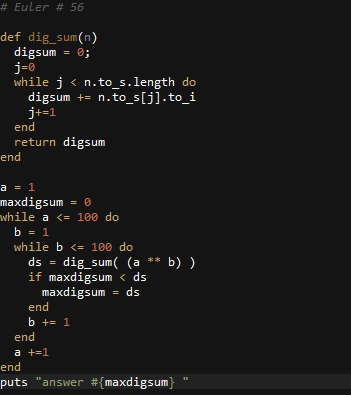# Ruby: Using Google Charts to Graph Chicago Crime Data

Recently I decided to visualize some of Chicago’s Crime Data.  I used ruby to parse and manicure it into JSON, and Google Charts to display it.

The Data is from : https://data.cityofchicago.org/

The data was saved in CSV format on my local C: Drive
Local Path : C:\ChicagoCrime\CHICAGOCRIME.csv
Data chosen ranges from 8/8/2012 to 8/8/2013

Ruby Code used to create the JSON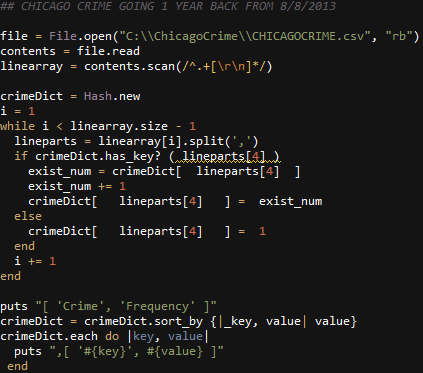# Ruby: Project Euler Problem # 23

http://projecteuler.net/problem=23

Non-abundant sums

A number n is called deficient if the sum of its proper divisors is less than n and it is called abundant if this sum exceeds n.

As 12 is the smallest abundant number, 1 + 2 + 3 + 4 + 6 = 16, the smallest number that can be written as the sum of two abundant numbers is 24. By mathematical analysis, it can be shown that all integers greater than 28123 can be written as the sum of two abundant numbers. However, this upper limit cannot be reduced any further by analysis even though it is known that the greatest number that cannot be expressed as the sum of two abundant numbers is less than this limit.

Find the sum of all the positive integers which cannot be written as the sum of two abundant numbers.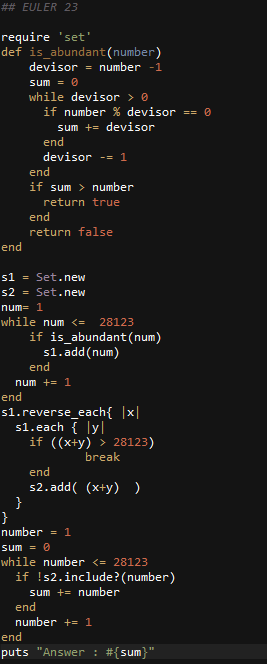# Ruby: Project Euler Problem # 24

http://projecteuler.net/problem=24

Lexicographic permutations

A permutation is an ordered arrangement of objects. For example, 3124 is one possible permutation of the digits 1, 2, 3 and 4. If all of the permutations are listed numerically or alphabetically, we call it lexicographic order.
What is the millionth lexicographic permutation of the digits 0, 1, 2, 3, 4, 5, 6, 7, 8 and 9?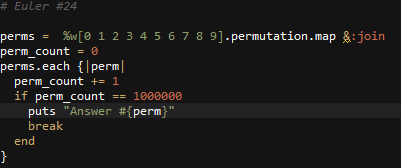# Ruby: Project Euler Problem # 99

http://projecteuler.net/problem=99

Largest exponential

Comparing two numbers written in index form like 211 and 37 is not difficult, as any calculator would confirm that 211 = 204837 = 2187.

However, confirming that 632382518061519432525806 would be much more difficult, as both numbers contain over three million digits.

Using base_exp.txt , a  text file containing one thousand lines with a base/exponent pair on each line, determine which line number has the greatest numerical value.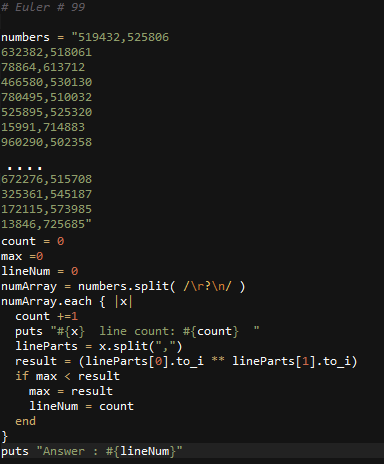# Ruby: Project Euler Problem # 97

http://projecteuler.net/problem=97

Large non-Mersenne prime

The first known prime found to exceed one million digits was discovered in 1999, and is a Mersenne prime of the form 269725931; it contains exactly 2,098,960 digits. Subsequently other Mersenne primes, of the form 2p1, have been found which contain more digits.
However, in 2004 there was found a massive non-Mersenne prime which contains 2,357,207 digits: 2843327830457+1.
Find the last ten digits of this prime number.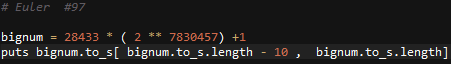# Ruby: Project Euler Problem # 58

http://projecteuler.net/problem=58

Spiral primes

Starting with 1 and spiralling anticlockwise in the following way, a square spiral with side length 7 is formed.

37 36 35 34 33 32 31
38 17 16 15 14 13 30
39 18  5  4  3 12 29
40 19  6  1  2 11 28
41 20  7  8  9 10 27
42 21 22 23 24 25 26
43 44 45 46 47 48 49

It is interesting to note that the odd squares lie along the bottom right diagonal, but what is more interesting is that 8 out of the 13 numbers lying along both diagonals are prime; that is, a ratio of 8/1362%.
If one complete new layer is wrapped around the spiral above, a square spiral with side length 9 will be formed. If this process is continued, what is the side length of the square spiral for which the ratio of primes along both diagonals first falls below 10%?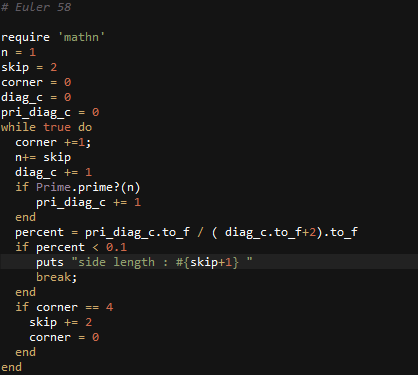# Ruby: Solution to Project Euler Problem # 53

http://projecteuler.net/problem=53

Combinatoric selections

There are exactly ten ways of selecting three from five, 12345:
123, 124, 125, 134, 135, 145, 234, 235, 245, and 345
In combinatorics, we use the notation, 5C3 = 10.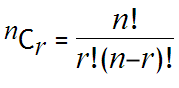where r n, n! = n(n1)…321, and 0! = 1.

How many, not necessarily distinct, values of  nCr, for 1n100, are greater than one-million?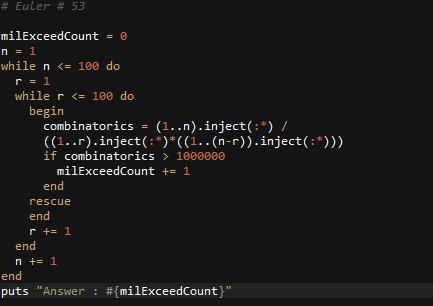# Ruby: Solution to Project Euler Problem # 56

http://projecteuler.net/problem=56

Powerful digit sum

A googol (10100) is a massive number: one followed by one-hundred zeros; 100100 is almost unimaginably large: one followed by two-hundred zeros. Despite their size, the sum of the digits in each number is only 1.

Considering natural numbers of the form, ab, where a, b100, what is the maximum digital sum?